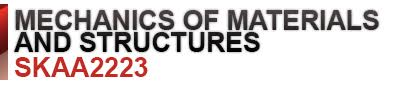## Topic outline

•### GeneralLecturer : SHEK POI NGIAN Semester : Semester 2 2014/2015

Synopsis :

This is a subject of the basic theory of the fundamental principles of mechanics of materials. Students will be able to incorporate these basic fundamentals into application of the basic design of simple structures . It will assure them of the concepts of stress and strain, plane-stress transformation, shear force and bending moment, stresses in beams, analysis of statically determinate plane and space trusses, deflections of beams, columns, and torsion. At the end of the course, the students should be able to solve numerous problems that depict realistic situations encountered in engineering practice. The students will also be able to develop and master the skills of reducing any such problem from its physical description to a model or symbolic representation to which the principles may be applied.This work, SKAA2223 Mechanics of Materials And Structures by Shek Poi Ngian is licensed under a Creative Commons Attribution-NonCommercial-ShareAlike 3.0 Unported License
•### Topic 1

TOPIC 1 STRESS AND STRAIN

• Introduction

• Types of forces

• Stress and strain

• Linear elasticity and Hooke`s law

• Normal stress and normal strain relationship

• Poisson’s ratio

• Shear stress and shear strain relationship

• Deflection of axially loaded member

• Composite bar

• Thermal stress

•### Topic 2

TOPIC 2 SHEAR FORCE AND BENDING MOMENT
• Introduction

• Types of beams and supports

• Shear force and bending moment

• Shear-force diagrams and Bending-moment diagrams

• Types of statically determinate frames

• Shear force and bending moment diagrams for frames

•### Topic 3

TOPIC 3 STRESSES IN BEAMS
• Introduction

• Bending stresses in beam

• Bending stress distribution

• Shear stresses in beam

• Shear stress distribution

•### Topic 4

TOPIC 4 STRESS TRANSFORMATION
• Introduction

• General equations for plane stress

• Stresses for inclined surface

• Principal stresses and Maximum shear stresses

• Mohr’s circle for plane stress

•### Topic 5

TOPIC 5 STATICALLY DETERMINATE PLANE TRUSSES
• Introduction

• Methods to determine member forces:

• Method of Joint

• Method of Sections

• Zero force member

• Virtual Work Method to determine displacements

•### Topic 6

TOPIC 6 STATICALLY DETERMINATE SPACE TRUSSES
• Introduction

• Analysis of space trusses

•### Topic 7

TOPIC 7 DEFLECTIONS OF BEAMS
• Introduction

• Calculation of slope and deflection using:Double Integration method

• Macaulay’s functions

• Moment area method

• Virtual work method or Unit Load method

•### Topic 8

TOPIC 8 COLUMN
• Introduction

• Euler’s theory

•